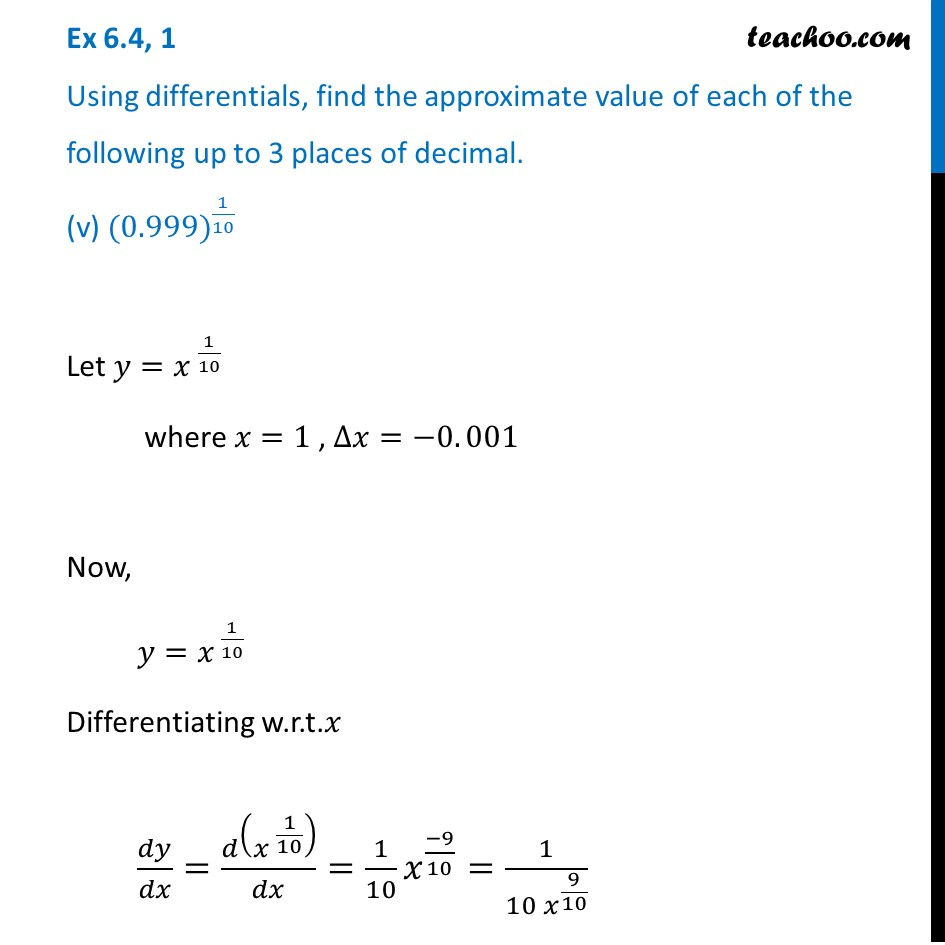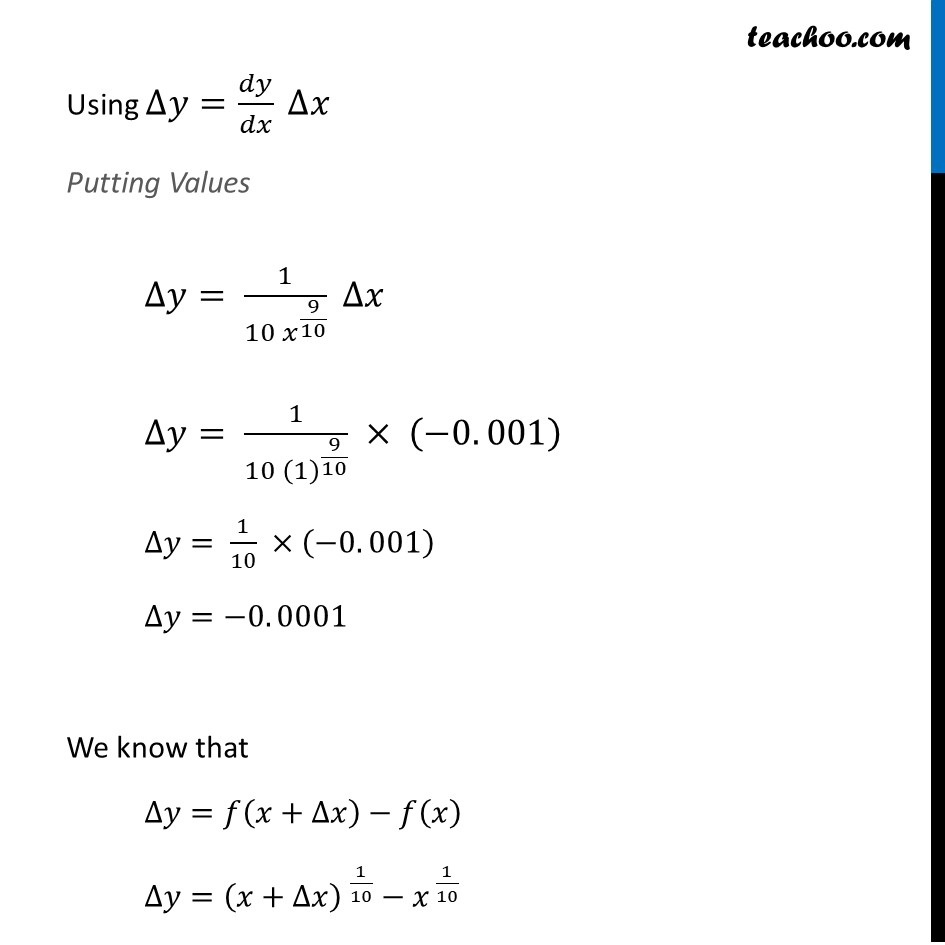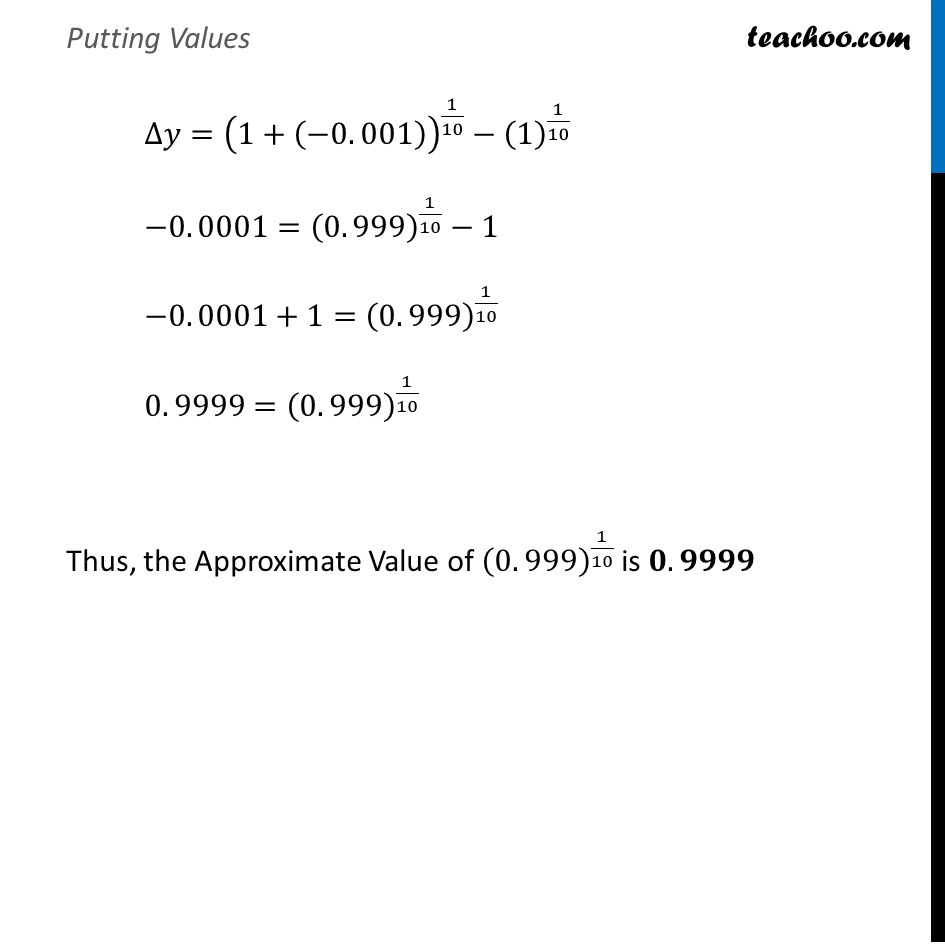Approximations (using Differentiation)

Chapter 6 Class 12 Application of Derivatives
Serial order wiseLearn in your speed, with individual attention - Teachoo Maths 1-on-1 Class

### Transcript

Question 1 Using differentials, find the approximate value of each of the following up to 3 places of decimal. (v) 〖(0.999)〗^(1/10)Let 𝑦=〖𝑥 〗^(1/10) where 𝑥=1 , ∆𝑥=−0. 001 Now, 𝑦=𝑥^( 1/10) Differentiating w.r.t.𝑥 𝑑𝑦/𝑑𝑥=𝑑(𝑥^( 1/10) )/𝑑𝑥=1/10 𝑥^((−9)/10)=1/(10〖 𝑥〗^(9/10) ) Using ∆𝑦=𝑑𝑦/𝑑𝑥 ∆𝑥 Putting Values ∆𝑦= 1/(10〖 𝑥〗^(9/10) ) ∆𝑥 ∆𝑦= 1/(10 (1)^(9/10) ) × (−0. 001) ∆𝑦= 1/10 ×(−0. 001) ∆𝑦=−0. 0001 We know that ∆𝑦=𝑓(𝑥+∆𝑥)−𝑓(𝑥) ∆𝑦=〖(𝑥+∆𝑥) 〗^(1/10)−𝑥^( 1/10) Putting Values ∆𝑦=(1+(−0. 001))^(1/10)−(1)^(1/10) −0. 0001=(0. 999)^(1/10)−1 −0. 0001+1=(0. 999)^(1/10) 0. 9999=(0. 999)^(1/10) Thus, the Approximate Value of (0. 999)^(1/10) is 𝟎. 𝟗𝟗𝟗𝟗